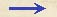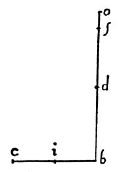Discorsi Propositions 3/13-pr-06 Discorsi Proposition3/13-pr-06{305} PROBLEMA, PROPOSITIO XIII. {305} PROBLEM. PROPOSITION XIIIEx datis semiparabolarum amplitudinibus, in praecedenti tabula digestis, retentoque communi impetu quo unaquaeque describitur, singularum semiparabolarum altitudines elicere. From the amplitudes of semi-parabolas given in the preceding table to find the altitudes of each of the parabolas described with the same initial speed. Sit amplitudo data bc; impetus vero, qui semper idem intelligatur, mensura sit ob, {1} aggregatum nempe altitudinis et sublimitatis: reperienda est ac distinguenda ipsamet altitudo; quod quidem tunc consequemur, cum bo ita divisa fuerit, ut rectangulum sub eius partibus contentum aequale sit quadrato {10} dimidiae amplitudinis bc.Incidat talis divisio in f; et utraque ob, bc secetur bifariam in d, i.Est igitur quadratum ib aequale rectangulo bfo; quadratum vero do aequatur eidem rectangulo cum quadrato fd: si igitur ex quadrato do auferatur quadratum bi, quod rectangulo bfo est aequale, remanebit quadratum fd, cuius latus df additum lineae bd dabit quaesitam altitudinem bf.Componitur itaque sic ex datis:Ex quadrato dimidiae bo notae aufer quadratum bi, pariter notae; residui sume radicem quadratam, quam adde notae db, et habebis altitudinem {20} quaesitam bf. Let bc denote the given amplitude; (Condition 3/10-th-07-cor) and let ob, the sum of the altitude and sublimity, be the measure of the initial speed which is understood to remain constant. Next we must find and determine the altitude, which (Condition 3/05-pr-02-cor) we shall accomplish by so dividing ob that the rectangle contained by its parts shall be equal to the square of half the amplitude, bc. Let f denote this point of division and d and i be the middle points of ob and bc respectively. The square of ib is equal to the rectangle bf.fo; but the square of do is equal to the sum of the rectangle bf.fo and the square of fd. If, therefore, from the square of do we subtract the square of bi which is equal to the rectangle bf.fo, there will remain the square of fd. The altitude in question, bf, is now obtained by adding to this length, fd, the line bd. The process is then as follows: From the square of half of bo which is known, subtract the square of bi which f is also known; take the square root of the remainder and add to it the known length db; then you have the required altitude, bf. Exemplum.Invenienda sit altitudo semiparabolae ad elevationem gr. 55 descriptae.Amplitudo, ex praecedenti tabula, est 9396; eius dimidium est 4698; quadratum ipsius 22071204; hoc dempto ex quadrato dimidiae bo, {306} quod semper idem est, nempe 25000000, residuum est 2928796, cuius radix quadrata 1710 proxime.Haec dimidiae bo, nempe 5000, addita, exhibet 6710; tantaque est altitudo bf. Example. To find the altitude of a semi-parabola described with an angle of elevation of 55°. From the preceding table the amplitude is seen to be 9396, of which the half is 4698, and the square 22071204. When this is subtracted from the square of the half of bo, which is always 25,000,000, the remainder is 2928796, of which the square root {306} is approximately 1710. Adding this to the half of bo, namely 5000, we have 6710 for the altitude of bf.Discorsi Propositions 3/13-pr-06 Discorsi Proposition3/13-pr-06